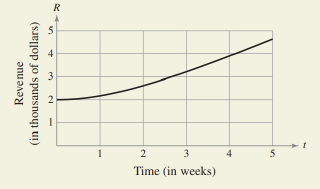Chapter 6.3, Problem 46E### Calculus: An Applied Approach (Min...

10th Edition
Ron Larson
ISBN: 9781305860919

#### Solutions

Chapter
Section### Calculus: An Applied Approach (Min...

10th Edition
Ron Larson
ISBN: 9781305860919
Textbook Problem
1 views

# HOW DO YOU SEE IT? The graph shows the weekly revenue (in thousands of dollars) for a company.Time (in weeks)(a) Which gives a more accurate approximation of the total weekly revenue for the first 4 weeks using the Trapezoidal Rule, n =   8   o r   n =   16 ?(b) Which gives a more accurate approximation of the total weekly revenue for the first 4 weeks, the Trapezoidal Rule with n = 8 or Simpson’s Rule with n = 8?

(a)

To determine

Weather n=8 or n=16 gives more accurate approximation using the Trapezoidal Rule, of total weekly revenue for the first four weeks for the graph shows the weekly revenue (in thousands of dollars) for a company.Explanation

Given Information:

The graph shows the weekly revenue (in thousands of dollars) for a company.

Formula used:

Trapezoidal Rule:

If f is continuous on [a,b] and n is an even integer, then

abf(x)dx,n=(ba2n)[f(x0)+2f(x1)+2f(x2)+2f(x3<

(b)

To determine

Weather n=8 of Trapezoidal Rule or n=8 Simpson’s Rule gives more accurate approximation for the first four weeks for the graph shows the weekly revenue (in thousands of dollars) for a company,### Still sussing out bartleby?

Check out a sample textbook solution.

See a sample solution

#### The Solution to Your Study Problems

Bartleby provides explanations to thousands of textbook problems written by our experts, many with advanced degrees!

Get Started

#### solve the equation by using the quadratic formula. 135. 4x2 + 5x 6 = 0

Applied Calculus for the Managerial, Life, and Social Sciences: A Brief Approach

#### 0 1 2 ∞

Study Guide for Stewart's Single Variable Calculus: Early Transcendentals, 8th

#### The area of the region bounded by , , and r = sec θ is:

Study Guide for Stewart's Multivariable Calculus, 8th